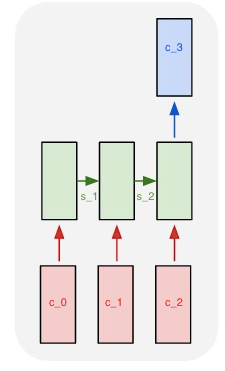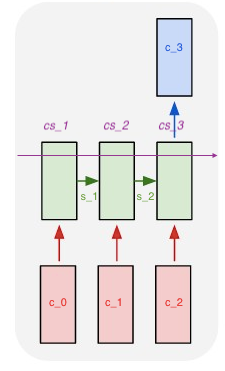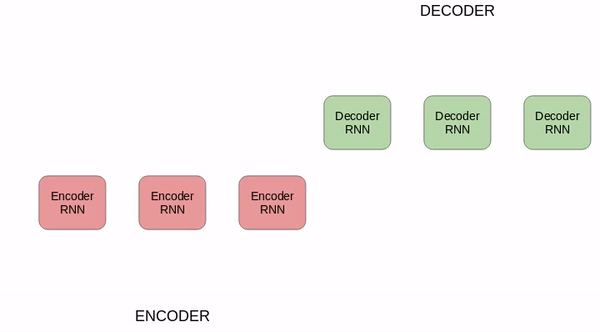# GPT-3, RNNs and All That: A Deep Dive into Language Modeling

ModelingNLP/Text Analyticsposted by ODSC Community March 7, 2022

As I’ve been working on Chai I’ve been exposed to large language models (LLMs), something I didn’t really know anything about previously....As I’ve been working on Chai I’ve been exposed to large language models (LLMs), something I didn’t really know anything about previously. In this article, I’ll summarise everything I have since learned on the subject. We’ll go from the very simple (what researchers were doing 40-ish years ago) to the state of the art, staying at a big picture level. The idea is not to get the details of the math right, but rather to be able to give a good “by and large” explanation of what is going on under the hood in these language models.

## What are we even modeling?

It’s easy to say “we train a model to understand language”, but what does that mean? One simple thing this can mean in practice is “given a sequence of characters, predict the most likely next character”. So, for example, we could train a model on a corpus of English words, then as input, we’d give it the phrase “Foo” and hopefully it would output the character “d” (or “l”) and we’d have the word “Food” or “Fool”. These sorts of models are Character-Level language models. Another thing it could mean is “given a sequence of words, predict the most likely next word”. This is the sort of problem large language models (LLMs) are used to solve.

## Toy problem: training a model to say hello

A super simple language modeling problem we could imagine is this: imagine we only knew four letters: H, E, L, and O. If we train a model on the training sequence “HELLO” we would expect that if we gave it as input “HE” it would output “L”, if we gave it “HELL” if would output “O”.

More formally we can write this as: what character c_n maximises the probability P(c_n | c_{n-1},….,c_0)?

In the above example, c_n is the letter “O”.

## How do you do math with words?

Neural networks are basically big calculus machines doing loads of partial derivatives (for back-propagation) and applying non-linear functions (e.g. tanh or sigmoid). But how do you do calculus with letters? You don’t. Instead, you use word embeddings, which means a schema for turning a character into a vector. The simplest of these methods is 1-to-k encoding: In our little example above our vocabulary is [H,E,L,O] so the letter H gets encoded as the [1,0,0,0] vector, the letter E as [0,1,0,0] etc… Ok, now we’ve turned characters into vectors, and we can definitely feed vectors into neural networks (this is the input layer).

## Multi-Layer Perceptrons applied to NLP

Your training data is a corpus of words (e.g. a book). The set of words in that corpus is your vocabulary. Say the book just says “eat the pizza eat the pizza eat the pizza”. There are only 3 words in your book: [“eat”, “the”, “pizza”]. Each of these words has its own embedding (or encoding): [1,0,0] is “eat”, [0,1,0] is “the”, and [0,0,1] is “pizza”. Notice that the length of each of these vectors is the size of our vocabulary (i.e. 3). So we give our multi-layer perceptron (MLP) an input layer of size 3. Then we can put as many hidden layers as we like, and our output layer must have size 3 as well. Then it’s the usual story: you initialize your network with random weights and biases, train your network example by example (encoded word by encoded word), calculate the loss, adjust the parameters with back-propagation, etc.

If you’re a bit shaky on how MLPs work, take a look at 3blue1brown’s video series on this, I’ve never seen a clearer explanation. The cool thing we’ve learned here is that you can use this technique to predict the next word in a sentence — so in principle this is enough to build a chat-bot.

Limitation of MLPs when applied to NLP: they take in a fixed size vector input and output a fixed size vector output. So in the context of predicting text, we can give them one word and they’ll output one more word. But what if I want to give them a sentence and get them to output the next word? Can’t do that, we can just give it the last word. This means it won’t be able to take into account the context from the previous words in the sentence.

Finally, we gave the simplest example of how to encode a word: our 1-to-k encoding. Clearly, we could have encoded the information in other ways. People use word2vec or gloVe but how they work isn’t too important, the cool thing is: take a word (or a character) → turn it into a vector → now you can do machine learning with words!

## Why Recurrent Neural Networks (RNNs) are more appropriate

The key thing that makes RNNs more exciting than MLPs for language modeling is that they allow us to deal with sequences of vectors. So we can input a sentence, which is a sequence of vector representations for words, and get an output vector.

Let’s limit ourselves to the case where we want to give a sequence of inputs (e.g. a list of vectors representing a sentence) and get a single output.

Instead of learning a set of weights and biases between layers to produce an output the way MLPs do, RNNs also learn a state vector. The output of the RNN is a function of this state vector. When we input a sequence of vectors to the model, this state vector gets passed along. So say we give a sequence of vectors (representing words) to the model and we want to output a single vector (representing the most likely next word).The c_i are the words (vectorized) that are input to the model except for c_3 which is the output. The s_i are the state vectors that get passed along. At each step the model can produce an output, these have been removed for all but the last step because we are only interested in building up the relevant state vector to predict c_3. Source: Andrej Karpathy’s blog.

Say we input three words and want a single word output. We input the first vector (c_0) in the sentence to the model, which updates its state vector to s_1. Then we input the next vector c_1, which updates the state vector to c_2. And do that one more time. The output of the model is a function of this final input, the state vector of the previous pass (which itself is a function of the first vector — so there is some memory of the start of the sentence) and a matrix of weights learned during training. And there we have it. At each pass, we are basically doing the same thing as we would with an MLP, but we’re also updating this state vector and passing it along.

If you want to give the model a lot of context, you need to give it a long input sequence of vectors. But this means that you’ll have many steps in your RNN.

The way weights and biases get updated is computed through the back-propagation algorithm. The issue is that the magnitude of the tweaks made in one layer’s weights and biases is proportional to the product of the derivatives of the loss function in all the layers between it and the output layer. These derivatives can be quite small for the activation functions used in RNNs (typically tanh), so their product tends to vanish. If the weights and biases in the first few layers receive very little updating because of this vanishing gradient, it means the network isn’t really learning how to use them.

So RNNs definitely do better at understanding context than MLPs, at least they have some short-term memory, but don’t expect them to remember something for too many layers because of the vanishing gradients.

## Long Short Term Memory Networks (LSTMs): tweaked RNNs that remember stuff for longer

LSTMs are a special kind of RNN designed to be able to handle long-term dependencies in a sequence of inputs. This means, in the context of a sentence input, that if the sentence is quite long, LSTMs will be better at taking into account the start of the sentence than a vanilla RNN like the one we described would.

The key innovation of the LSTM is the “cell state”, basically this is another state vector like the s_i from our previous diagram, but it gets updated differently. There are functions called “gates” that control, at each step in the sequence of inputs, what gets forgotten from the cell state, and what gets added. There are two gates: the “forget gate” and the “input gate”. They get to remove and add, respectively, information to the cell state. The exact mechanism behind these things is not particularly complicated or interesting, as is often the case with machine learning it’s just some funky combination of sigmoids and tanh function applications that you apply to vectors and the result is the new cell state.The cs_i represent the cell state, like a conveyor belt of information that gets selectively updated at each new input from the sequence. Source: image from Andrej Karpathy’s blog (edited by the author).

## Drawbacks to using RNNs/LSTMs

LSTMs and vanilla-RNNs get some pretty good performance at language modeling tasks (as well as some other very cool stuff like playing StarCraft). But one of the big drawbacks is that it takes forever to train them. Because of the sequential nature of their architecture, it is not possible to parallelize the training of these models. And since with deep learning it is usually the case that (when done well) more data, more layers and more training lead to better performance it is frustrating to not be able to speed up the training of massive RNNs.

I learned a few things as a software developer, and one of them was: when code is slow, parallelize the f*** out of it! There was a case of a PhD student I heard of in the physics department at Cambridge who took a bit of software that would take weeks to run and he made it his mission to speed it up. Through massive parallelization he managed to bring it down to a few hours.

## Encoder-Decoder networks

We’re going to stray into the world of machine translation for a bit (this is what transformers were initially invented to be used for). Say you want to translate a sentence from German into English. You can do this with two RNNs, one “Encoder” and one “Decoder”. The Diagram below makes it clearer:First RNN (in red) encodes the information from the sequence of german words. The second RNN (in green) decodes this information into English. The H_i are the state vectors (“hidden states”), <SOS> means “Start of Sequence”. Source: this paper by Bezerra et al.

## Attention mechanisms

When we go from the encoder to the decoder we can either: give the decoder network the final hidden state, or we could give it a weighted sum of all the hidden states. The latter is known as an “attention mechanism”. As usual, funky sounding name but a pretty simple idea underlying it. As far as I can tell, other attention mechanisms are basically just more complicated versions of “do a weighted mean of the hidden states”.

Ok, the last two paragraphs were pretty simple but this was necessary context to understand the transformer.

## Transformer models

This model architecture is considered to be the state of the art in NLP. They adopt an encoder-decoder structure but neither of these are RNNs, instead, they are a combination of MLPs and attention mechanisms.

At a high level this is how a transformer works: take all the vectors in your sequence, compute the encoder states for all of them using “multi-head” attention and an MLP. You don’t need to know the encoder state of the first element in the sequence to compute the encoder state of subsequent elements in the sequence. This is very neat because it helps massively speed up the training of models through parallel processing.

What exactly “multi-head attention” is doesn’t matter too much for a rough understanding of what’s going on. Basically, the thing is calculating how much each other word (vector) in the sequence is relevant to the input being processed.

Then all the encoded states are passed into the decoder network, these are then processed sequentially (so this can’t actually be parallelized) to produce the output sequence.

## GPT-3: an example of a transformer

According to Wikipedia, GPT-3 is “an autoregressive language model”. Autoregressive is just a fancy word to say this: commonly known models (like linear regression) are “regressive”, i.e. they use a bunch of predictor variables to predict the future value of the target variable. An autoregressive model, by contrast, only uses the previous value of the target variable to predict the future value. So if you were modeling the price of a stock for example we’d say your model is “autoregressive” if you only used the past values of the price of that stock to predict the future value. Your model would be “regressive” if instead, you used other information, for example, the P/E ratio. So all the models we’ve looked at in this article are autoregressive.

And there we have it! Hopefully, this article helped you get a high-level understanding of what’s been going on in language modeling for the last few decades and these things work: we went from MLPs, to RNNs, to LSTMs, to Transformers.

## Sources:

Article originally posted here. Reposted with permission.

Thomas Rialan: Co-Founder of chai.ml. University of Cambridge Astrophysicist. Former pro-gambler.## ODSC Community

The Open Data Science community is passionate and diverse, and we always welcome contributions from data science professionals! All of the articles under this profile are from our community, with individual authors mentioned in the text itself.

1